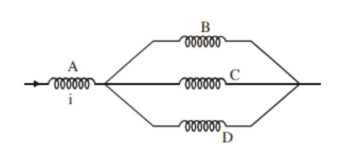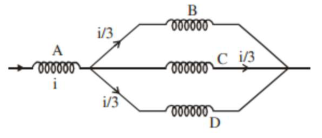# Four identical long solenoids A, B, C and DQuestion:

Four identical long solenoids $\mathrm{A}, \mathrm{B}, \mathrm{C}$ and $\mathrm{D}$ are connected to each other as shown in the figure. If the magnetic field at the center of $\mathrm{A}$ is $3 \mathrm{~T}$ the field at the center of $\mathrm{C}$ would be : (Assume that the magnetic field is confined with in the volume of respective solenoid).1. (1) $12 \mathrm{~T}$

2. (2) $6 \mathrm{~T}$

3. (3) $9 \mathrm{~T}$

4. (4) $1 \mathrm{~T}$

Correct Option: , 4

Solution:

(4)$\phi \propto \mathrm{i}$

$\Rightarrow \mathbf{B} \propto \mathrm{i}$

so, field at centre of $\mathrm{C}=\frac{3}{3}=1 \mathrm{~T}$